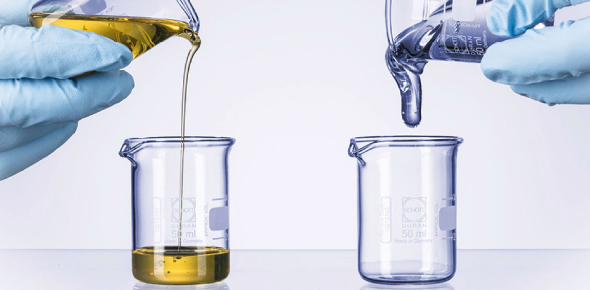# How Much Do You Know About Viscosity Of Fluids?

10 Questions | Total Attempts: 1843SettingsViscosity is a crucial parameter for measuring the flow of fluids, such as liquids, semi-solids, gases and even solids. It can be defined as quantification of the frictional force that arises between two adjacent layers of fluid that are in relative motion. This quiz has been created to test your knowledge and make you understand about concepts of the viscosity of fluids So, let's try out the quiz. All the best!

Related Topics
• 1.
What measures a material's resistance to flow?
• A.

Matter

• B.

Volume

• C.

Hardness

• D.

Tensile Strength

• E.

Viscosity

• 2.
• A.

The viscosity of the liquid increases.

• B.

The viscosity of the liquid stays the same.

• C.

The viscosity of the liquid decreases.

• D.

The temperature of a liquid does NOT raise.

• E.

• 3.
• A.

The molecules tend to form tight connections called "hydrogen connections".

• B.

Nothing happens.

• C.

The molecules tend to form loose conections called "hydogen bonds".

• D.

The temperatures raises.

• E.

The olive oil loses molecules.

• 4.
As you raise the temperature of a gas what will happen?
• A.

It becomes more resistant to flow.

• B.

It becomes less resistance to flow.

• C.

It turns into a solid.

• D.

It turns into a liquid.

• E.

Nothing happens

• 5.
When friction increases what else increases?
• A.

Matter

• B.

Density

• C.

Elasticity

• D.

Viscosity

• E.

Number of molecules

• 6.
Viscosity is determined mostly by what?
• A.

Density

• B.

The shape of the molecules the liquid.

• C.

What the liquid is specificaly.

• D.

Mass/Volume

• E.

Density*Volume

• 7.
Out the of the three liquids listed below, which is the most viscous?: 1. Ketchup 2. Vegtable oil 3. Water
• A.

2.Vegetable oil

• B.

3.Water

• C.

1.Ketchup

• 8.
• A.

When a solid, quickly flows out of its container.

• B.

Same definition as High-viscosity.

• C.

When a fluid, slowly empties from its container.

• D.

When a fluid quickly flows out of its container.

• E.

• 9.
Any substance that can flow is a
• A.

Liquid

• B.

Fluid

• C.

Gas

• D.

Water

• 10.
What principle is in effect when a ship displaces ocean water?
• A.

Archimedes'

• B.

Bernoulli's

• C.

Newton's Law of Motion

• D.

None